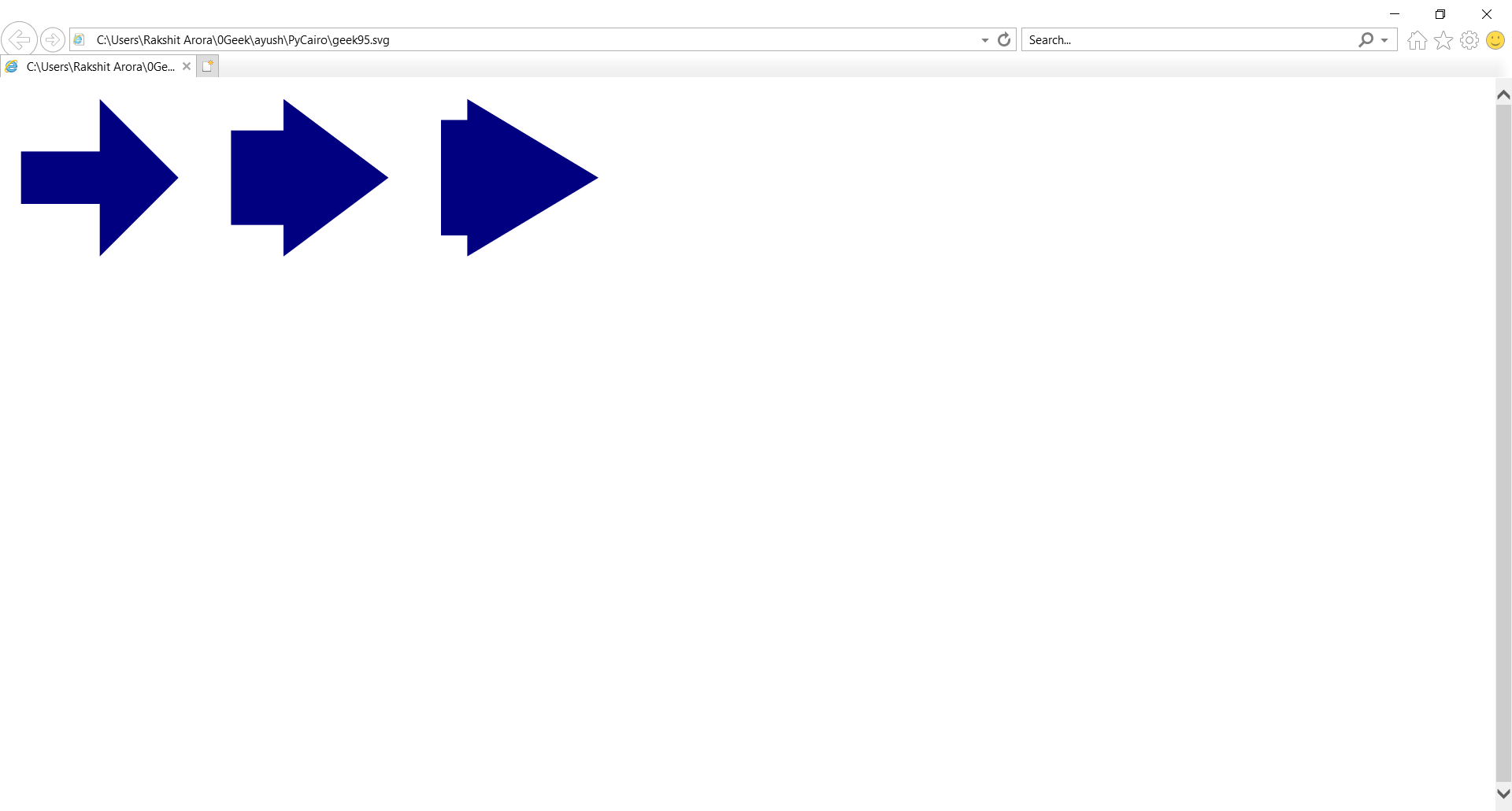GeeksforGeeks App
Open AppBrowser
Continue

# PyCairo – A simple arrow shape

In this article we will learn how we can draw a simple arrow shape using PyCairo in python.

PyCairo : Pycairo is a Python module providing bindings for the cairo graphics library.This library is used for creating SVG i.e vector files in python. The easiest and quickest way to open an SVG file to view it (read only) is with a modern web browser like Chrome, Firefox, Edge, or Internet Explorer—nearly all of them should provide some sort of rendering support for the SVG format.

SVG file is a graphics file that uses a two-dimensional vector graphic format created by the World Wide Web Consortium (W3C). It describes images using a text format that is based on XML. SVG files are developed as a standard format for displaying vector graphics on the web.

Steps of Implementation :

1. Import the Pycairo module.
2. Create an SVG surface object and add context to it.
3. Creating function for make arrow shape
4. Calling the function with parameters
5. Fill inside color of object using fill ( )

Below is the Implementation :

## Python3

 `# importing pycairo``import` `cairo` `# Creating function for make arrow shape`  `def` `arrow(context, x, y, width, height, a, b):``    ``context.move_to(x, y ``+` `b)``    ``context.line_to(x, y ``+` `height ``-` `b)``    ``context.line_to(x ``+` `a, y ``+` `height ``-` `b)``    ``context.line_to(x ``+` `a, y ``+` `height)``    ``context.line_to(x ``+` `width, y ``+` `height``/``2``)``    ``context.line_to(x ``+` `a, y)``    ``context.line_to(x ``+` `a, y ``+` `b)``    ``context.close_path()`  `    ``# creating a SVG surface``    ``# here geek95 is file name & 700, 700 is dimension``with cairo.SVGSurface(``"geek95.svg"``, ``700``, ``700``) as surface:` `    ``# creating a cairo context object for SVG surface``    ``# using Context method``    ``context ``=` `cairo.Context(surface)` `    ``context.set_source_rgb(``0``, ``0``, ``0.5``)``    ``# Call the function``    ``arrow(context, ``20``, ``20``, ``150``, ``150``, ``75``, ``50``)``    ``# Fill the color inside``    ``context.fill()` `    ``# Call the function``    ``arrow(context, ``220``, ``20``, ``150``, ``150``, ``50``, ``30``)``    ``# Fill the color inside``    ``context.fill()``    ``# Call the function``    ``arrow(context, ``420``, ``20``, ``150``, ``150``, ``25``, ``20``)``    ``# Fill the color inside``    ``context.fill()``    ``# Call the function``    ``arrow(context, ``70``, ``220``, ``75``, ``150``, ``0``, ``50``)``    ``# Fill the color inside``    ``context.fill()``   ``# stroke out the color and width property``    ``# context.stroke()` `  ``# printing message when file is saved``print``(``"File Saved"``)`

Output :My Personal Notes arrow_drop_up